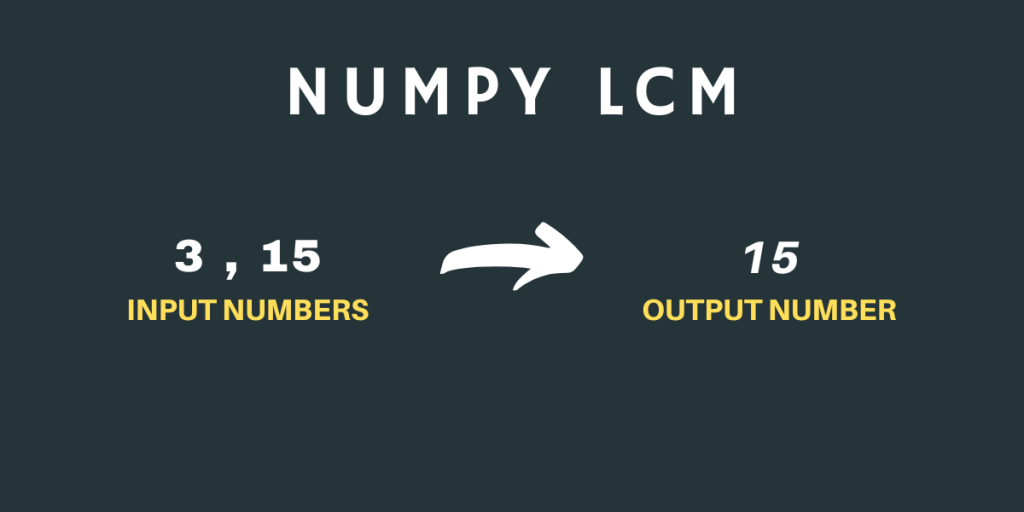# NumPy lcm – Returns the Lowest Common Multiple of two numbersHello readers! Welcome to another tutorial on NumPy Mathematical Functions. In this tutorial, we will be studying the NumPy lcm function in detail with examples.

You must have calculated the lcm(least common multiple) of two numbers in the Mathematics class. It was fun calculating it 🙂

Now, let us see how can we calculate the lcm using Python Programming Language. Let’s get started.

## What is NumPy lcm?

NumPy lcm is one of the mathematical functions of the NumPy Library that calculates the lcm of two input numbers. Here, the input numbers must be positive.

Let us look at the syntax of the function.

## Syntax of NumPy lcm

```numpy.lcm(x1 , x2)
```

Here, x1 and x2 can be single numbers as well as a NumPy array of Numbers.

Note: The numbers x1 and x2 cannot be floating point digits.

## Working with NumPy lcm

Let’s write some code to work on the numpy.lcm() method and see how to use it.

### NumPy lcm with Single Number

```import numpy as np

print("The lcm of 3 and 15 is:",np.lcm(3 , 15))

print("The lcm of 12 and 44 is:",np.lcm(12 , 44))

print("The lcm of 3 and 9 is:",np.lcm(3 , 9))

print("The lcm of 120 and 200 is:",np.lcm(120,200))
```

### Output

```The lcm of 3 and 15 is: 15
The lcm of 12 and 44 is: 132
The lcm of 3 and 9 is: 9
The lcm of 120 and 200 is: 600
```

The outputs are pretty obvious. Now, let’s see how can we calculate the lcm of two NumPy arrays.

### NumPy lcm with NumPy Array

```import numpy as np

a = np.array((12,44,78,144,10000))

b = np.array((24,54,18,120,100))

print("Input Arrays:\n",a,"\n",b)

print("The lcm values:\n",np.lcm(a , b))
```

### Output

```Input Arrays:
[   12    44    78   144 10000]
[ 24  54  18 120 100]
The lcm values:
[   24  1188   234   720 10000]
```

`numpy.lcm()` picks one element from each of the two NumPy arrays and calculates their lcm.

That was all about the NumPy lcm function, this function is easy to understand and simple to use. Stay tuned for more such articles.

## Reference

NumPy Documentation – NumPy lcm